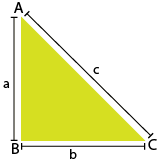## Objective

To verify the Pythagoras theorem by the Bhaskara method.

## What is Right triangle?### Fig. 1

• If one of the angles of a triangle is a right angle (90º), then the triangle is called a right-angled triangle, or simply a right triangle.
• In the given image, triangle ABC is a right triangle, where we have the base, the altitude, and the hypotenuse.
• Here BC is the base, AB is the altitude, and AC is the hypotenuse.
• The hypotenuse is the largest side and is opposite the right angle within the triangle.

## What is pythagoras theorem?

• The Pythagoras theorem, or the Pythagorean theorem, named after the Greek mathematician Pythagoras, states that:
• In any right triangle, the area of the square whose side is the hypotenuse (the side opposite to the right angle) is equal to the sum of the areas of the squares whose sides are the two legs (the two sides that meet at a right angle).
• This is usually summarised as follows:
• The square of the hypotenuse (longest side) of a right triangle is equal to the sum of the squares on the other two sides.
• For example, from Fig. (1), we can write the Pythagoras theorem in the form of an equation:
• a² + b² = c²
• where c is the length of the hypotenuse and
• a and b are the lengths of the other two sides of a right triangle.